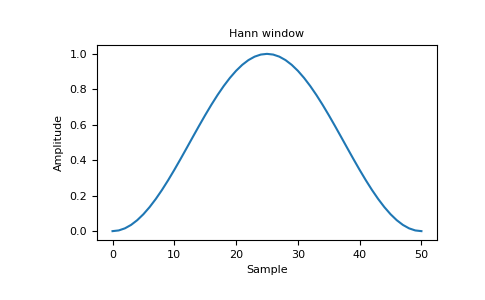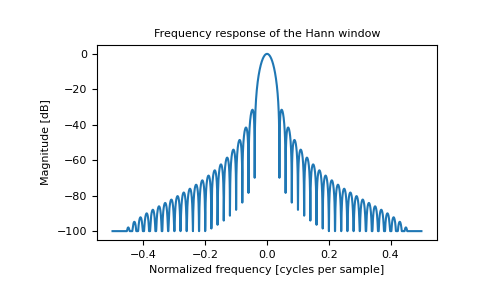Related Articles

# Hanning in Numpy | Python

• Last Updated : 22 Jul, 2021

The Hanning window is a taper formed by using a weighted cosine.

```Syntax:  numpy.hamming(M)

Parameters:
M :  Number of points in the output window.

Returns:  AN array```

The window, with the maximum value normalized to one (the value one appears only if M is odd).

Attention geek! Strengthen your foundations with the Python Programming Foundation Course and learn the basics.

To begin with, your interview preparations Enhance your Data Structures concepts with the Python DS Course. And to begin with your Machine Learning Journey, join the Machine Learning - Basic Level Course

Code #1:

 `import` `numpy as np`` ` ` ` `print``(np.hanning(``12``))`

Output:

```[ 0.          0.07937323  0.29229249  0.57115742  0.82743037  0.97974649
0.97974649  0.82743037  0.57115742  0.29229249  0.07937323  0.        ]
```

Code #2: Plotting the window and its frequency response (requires SciPy and matplotlib).

 `import` `numpy as np``import` `matplotlib.pyplot as plt``from` `numpy.fft ``import` `fft, fftshift`` ` `window ``=` `np.hanning(``51``)`` ` `plt.plot(window)``plt.title(``"Hann window"``)``plt.ylabel(``"Amplitude""``)``plt.xlabel(``"Sample"``)`` ` `plt.show()`

Output:For frequency:

 `import` `numpy as np``import` `matplotlib.pyplot as plt``from` `numpy.fft ``import` `fft, fftshift`` ` `window ``=` `np.hanning(``51``)``plt.figure()`` ` `A ``=` `fft(window, ``2048``) ``/` `25.5``mag ``=` `np.``abs``(fftshift(A))``freq ``=` `np.linspace(``-``0.5``, ``0.5``, ``len``(A))``response ``=` `20` `*` `np.log10(mag)``response ``=` `np.clip(response, ``-``100``, ``100``)`` ` `plt.plot(freq, response)``plt.title(``"Frequency response of Hanning window"``)``plt.ylabel(``"Magnitude [dB]"``)``plt.xlabel(``"Normalized frequency [cycles per sample]"``)``plt.axis(``"tight"``)``plt.show()`

Output:My Personal Notes arrow_drop_up